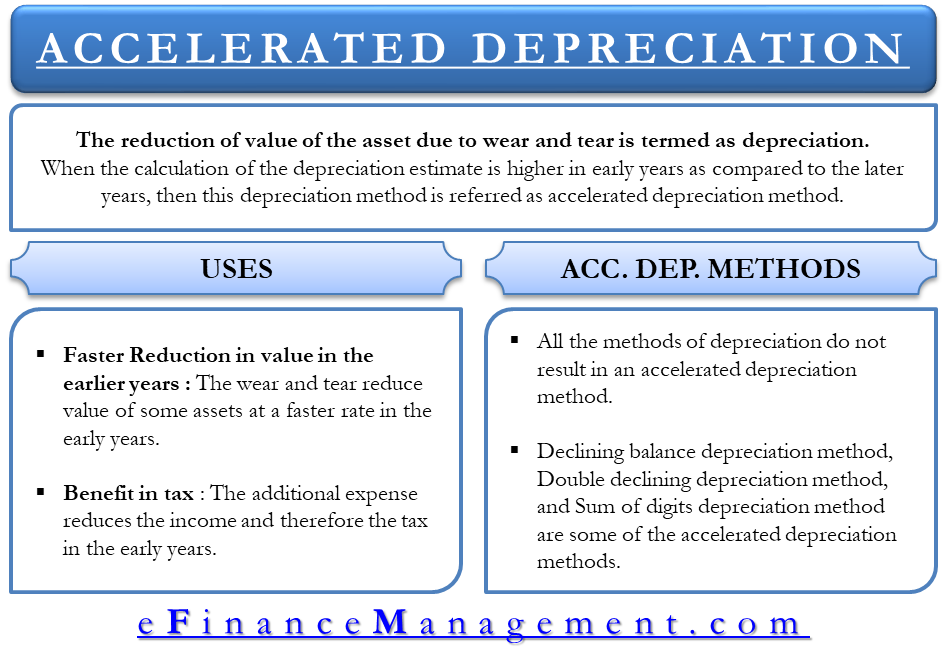data-full-width-responsive="true">

Accelerated Depreciation Method

The value of a fixed asset or long term assets reduces due to wear and tear. This reduction of value of the asset is depreciation. There are various methods like straight lime method, declining balance method, and units of the production method that helps in calculating the depreciation. The depreciation estimate for each year of the life of the asset will be different for each method. When the calculation of the depreciation estimate is higher in early years as compared to the later years, then the depreciation is known as accelerated and the method applied to calculate the depreciation is referred as accelerated depreciation method.

Benefits of Accelerated Depreciation Method

The business uses the accelerated depreciation method for the following key reasons:

Faster Reduction in Value in the Early Years

The wear and tear reduce the value of some assets at a faster rate in the early years, therefore the use of accelerated depreciation methods shows the actual wear and tear on the asset over its life.

Benefit in Tax

The additional expense reduces the income and therefore the tax in the early years. The drawback of this is that the business will look less profitable in the early years.

Types of Accelerated Depreciation Method

All the methods of depreciation do not result in an accelerated depreciation method. For example, the straight-line method. The straight line method calculates the depreciation which is the same each year.

The methods that produce higher depreciation expenses in the earlier years than in the following years are therefore considered as accelerated depreciation methods. Such methods are:

1. Declining balance depreciation method,
2. Double declining depreciation method, and
3. Sum of digits depreciation methodExample of Accelerated Depreciation Method

Suppose a business purchases an asset costing 40,000 having a scrap value of 2000 after 5 years of useful life. Then the accelerated depreciation for the first 5 years using the double declining balance method is calculated as:

Calculation as per Double Declining Method

Double Declining rate=2*1/assets useful life

Double Declining Rate= 2*1/5= 40%

1st Year=40%*40,000=16000

2nd Year=40%*24000=9600

3rd Year=40%*14400=5760

4th Year=40%*8640=3456

5th Year=40%*5184=2073 (approx. the salvage or scrap value).

This example shows that the depreciation is higher in the first year (16000) and declines in the following years. Therefore the double declining method is called an accelerated depreciation method.

Calculation as per Straight-line Method

Now, let’s calculate the depreciation using the straight-line method.

Straight line depreciation= (cost of asset- scrap or salvage value)/Useful life of the asset

Straight line depreciation=(40000-2000)/5= 7600

1st Year =7600

2nd Year =7600

———–

———–

5th Year =7600

In this calculation using the straight-line method, the depreciation for each year is the same. The depreciation dues not accelerate; therefore the straight line method is not an accelerated depreciation method.

When is this Method used by the Business?

The business uses the accelerated depreciation method when an asset will generate higher income in its early years. This helps the business to match the income generated by the asset to the expense of using the asset. The business also uses this method in case of the assets that are likely to dispose before the end of their life spans, like computers and motor vehicles.

Example

Let’s see the example with computers. The computer can have a useful life of 7 years, but it does not work well in the last two years. Let’s say it is barely operating. The management is using it until they can get the budget to purchase the new computer.

Does it make any sense to distribute the same amount of cost in the first year when the computer was brand new and giving its best performance and to the seventh years when it was barely working? The method of accelerated depreciation takes care of this difference. This method distributes a large amount of cost to the early years and smaller portions to the later years. This way the accelerated method follows the principle of matching by matching the benefits from the asset to its expenses. The business gets to benefit from computers in the early years than the later years. Thus, they realize more cost/ expense in the early years.

Last updated on : May 31st, 2019

** Disclaimer: This post may contain Affiliate Links marked as ** and we may earn a commission on sale.